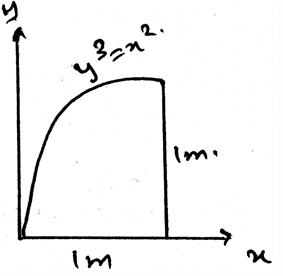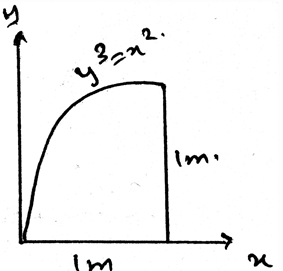Courses

# Test: Moment Of Inertia For Areas

## 15 Questions MCQ Test Engineering Mechanics - Notes, Videos, MCQs & PPTs | Test: Moment Of Inertia For Areas

Description
This mock test of Test: Moment Of Inertia For Areas for Mechanical Engineering helps you for every Mechanical Engineering entrance exam. This contains 15 Multiple Choice Questions for Mechanical Engineering Test: Moment Of Inertia For Areas (mcq) to study with solutions a complete question bank. The solved questions answers in this Test: Moment Of Inertia For Areas quiz give you a good mix of easy questions and tough questions. Mechanical Engineering students definitely take this Test: Moment Of Inertia For Areas exercise for a better result in the exam. You can find other Test: Moment Of Inertia For Areas extra questions, long questions & short questions for Mechanical Engineering on EduRev as well by searching above.
QUESTION: 1

### Whenever the distributed loading acts perpendicular to an area its intensity varies __________

Solution:

The load intensity is varying linearly in the structures. Thus the intensity is not varying parabolically nor is it cubically. It cannot be a vector also. Thus the intensity is linearly varied.

QUESTION: 2

### Determine the moment of inertia of the area about y-axis.Solution:

Parallel axis for any area is used to add the two mutually perpendicular moment of inertias for areas. It gives a moment of inertia perpendicular to the surface of the body. That is the moment of inertia perpendicular to the surface in considerance.

QUESTION: 3

### The calculation of the moment of the body due to the loadings involve a quantity called ____________

Solution:

The calculation of the moment of the body due to the loadings involve a quantity called moment of inertia. This is having much significance in the various fields in the engineering sector. The main types are the ‘I’ section structures which are being much used.

QUESTION: 4

Moment of Inertia is the integration of the square of the distance of the centroid and the del area along the whole area of the structure.

Solution:

The moment of inertia of the section is the integration of the square of the distance of the centroid and the del area along the whole area of the structure. This is having much significance in the various fields in the engineering sector. The main types are the ‘I’ section structures which are being much used.

QUESTION: 5

There is perpendicular axis theorem for the area.

Solution:

There is no perpendicular axis theorem for the area. In spite there is the theorem as parallel axis for any area. Thus we have the theorem which is used to add the two mutually perpendicular moment of inertias.

QUESTION: 6

What is parallel axis theorem and to whom it is applied?

Solution:

Parallel axis for any area is used to add the two mutually perpendicular moment of inertias for areas. It gives a moment of inertia perpendicular to the surface of the body. That is the moment of inertia perpendicular to the surface in considerance.

QUESTION: 7

The parallel axis theorem gives the moment of inertia ______________ to the surface of considerance.

Solution:

Parallel axis for any area is used to add the two mutually perpendicular moment of inertias for areas. It gives a moment of inertia perpendicular to the surface of the body. That is the moment of inertia perpendicular to the surface in considerance.

QUESTION: 8

The parallel axis theorem can add any angle varied moment of inertias to give the perpendicular moment of inertia.

Solution:

Parallel axis for any area is used to add the two mutually perpendicular moment of inertias for areas. It gives a moment of inertia perpendicular to the surface of the body. That is the moment of inertia perpendicular to the surface in considerance.

QUESTION: 9

The parallel axis theorem uses the ____________ of the distance.

Solution:

Parallel axis for any area is used to add the two mutually perpendicular moment of inertias for areas. It gives a moment of inertia perpendicular to the surface of the body. And uses the square of the distance from the axis of rotation.

QUESTION: 10

The distance in the parallel axis theorem is multiplied by ___________

Solution:

Parallel axis for any area is used to add the two mutually perpendicular moment of inertias for areas. It gives a moment of inertia perpendicular to the surface of the body. And uses the square of the distance from the axis of rotation multiplied by the area.

QUESTION: 11

One of the use of the centre of mass or centroid is as in the moment of inertia is that the net force acts at the ___________ of the loading body.

Solution:

In the moment of inertia calculations we see that the net force acts at the centroid of the loading body. That is if the loading system is in the form of the triangle then the at the distance 2 by 3 of the base the net force of the loading will act. And the load will be half the area of the loading.

QUESTION: 12

If the non-Uniform loading is of the type of parabola then for calculating the moment of inertia for areas?

Solution:

The net force will act at the centroid of the parabola. Whether it be a parabola or the cubic curve the centroid is the only point at which the net force act. Force can’t be acted horizontally if the loading is vertical. Hence whatever be the shape of the loading, the centroid is the point of action of net force. Thus the use of centroid.

QUESTION: 13

If any external force also is applied on the structure and we are determining the moment of inertia then what should we consider?

Solution:

The external forces are treated differently. They are not added by the force of the distributed loading. That is the force not only acts at the centroid always. It can be shifted also. Depending on the external forces. Thus the use of centroid or centre of mass.

QUESTION: 14

The body is sometimes acted by two or three force members and we need to find the moment of inertia for the same. The difference between the two and the three force members is:

Solution:

The definition of the two force member only defines that the forces are being acted on the two points on the body. So does is the definition of the three forces members. The points of action of the three forces are three.

QUESTION: 15

Determine the moment of inertia of the area about x-axis.Solution:

Parallel axis for any area is used to add the two mutually perpendicular moment of inertias for areas. It gives a moment of inertia perpendicular to the surface of the body. That is the moment of inertia perpendicular to the surface in considerance.